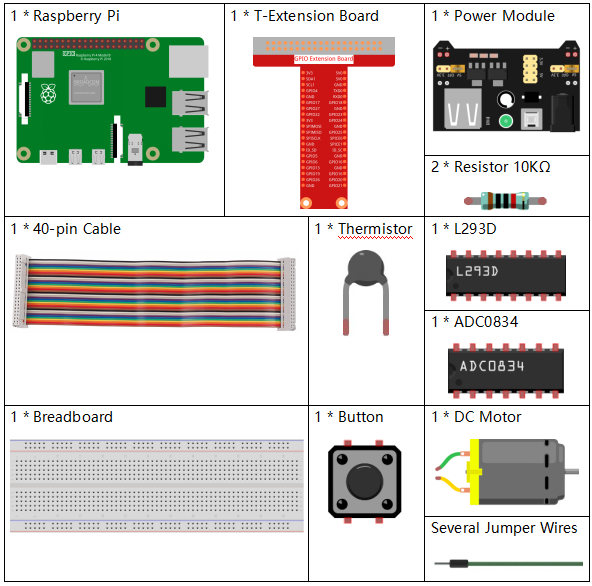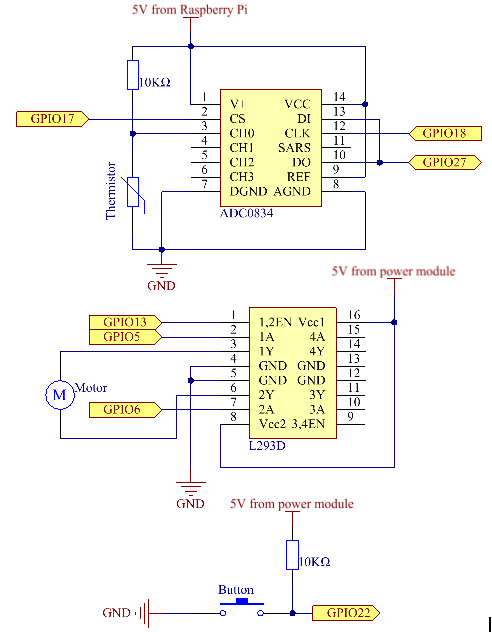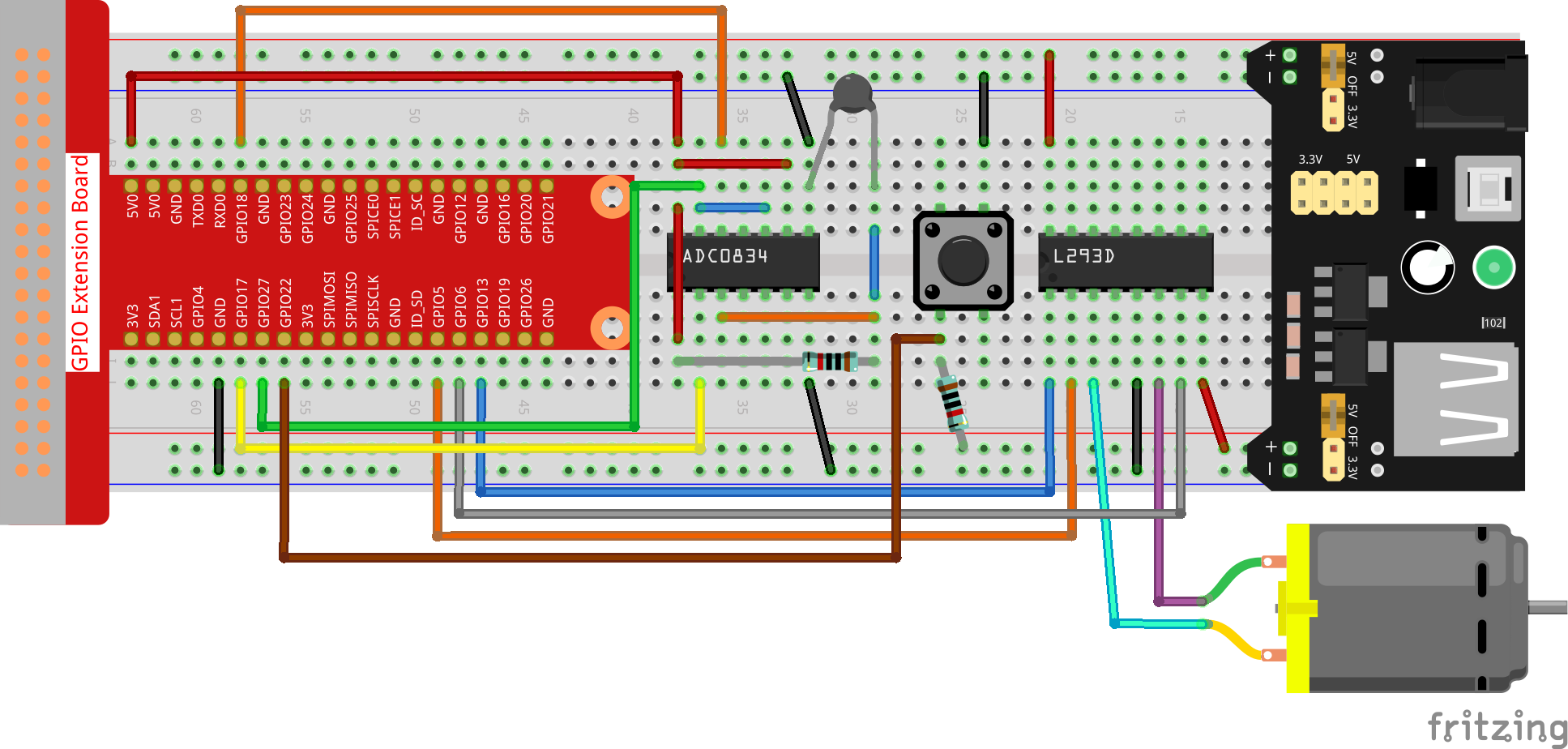# 3.1.4 Smart Fan¶

## Introduction¶

In this project, we will use motors, buttons and thermistors to make a manual + automatic smart fan whose wind speed is adjustable.

## Required Components¶

In this project, we need the following components.Name

ITEMS IN THIS KIT

Raphael Kit

337

Raphael Kit

COMPONENT INTRODUCTION

GPIO Extension Board

Jumper Wires

Resistor

Power Supply Module

-

Thermistor

L293D

-

-

Button

DC Motor

## Schematic Diagram¶

 T-Board Name physical wiringPi BCM GPIO17 Pin 11 0 17 GPIO18 Pin 12 1 18 GPIO27 Pin 13 2 27 GPIO22 Pin 15 3 22 GPIO5 Pin 29 21 5 GPIO6 Pin 31 22 6 GPIO13 Pin 33 23 13## Experimental Procedures¶

Step 1: Build the circuit.Note

The power module can apply a 9V battery with the 9V Battery Buckle in the kit. Insert the jumper cap of the power module into the 5V bus strips of the breadboard.Step 2: Get into the folder of the code.

```cd ~/raphael-kit/c/3.1.4/
```

Step 3: Compile.

```gcc 3.1.4_SmartFan.c -lwiringPi -lm
```

Step 4: Run the executable file above.

```sudo ./a.out
```

As the code runs, start the fan by pressing the button. Every time you press, 1 speed grade is adjusted up or down. There are 5 kinds of speed grades: 0~4. When set to the 4th speed grade and you press the button, the fan stops working with a 0 wind speed.

Once the temperature goes up or down for more than 2℃, the speed automatically gets 1-grade faster or slower.

Note

If it does not work after running, or there is an error prompt: "wiringPi.h: No such file or directory", please refer to Install and Check the WiringPi.

## Code¶

```#include <wiringPi.h>
#include <stdio.h>
#include <softPwm.h>
#include <math.h>

typedef unsigned char uchar;
typedef unsigned int uint;

#define MotorPin1   21
#define MotorPin2   22
#define MotorEnable 23
#define BtnPin      3

{
uchar i;
uchar dat1=0, dat2=0;
int sel = channel > 1 & 1;
int odd = channel & 1;

// Start bit
//Single End mode
// ODD
//Select

for(i=0;i<8;i++)
{

}

for(i=0;i<8;i++)
{
}

return(dat1==dat2) ? dat1 : 0;
}

int temperture(){
unsigned char analogVal;
double Vr, Rt, temp, cel, Fah;
Vr = 5 * (double)(analogVal) / 255;
Rt = 10000 * (double)(Vr) / (5 - (double)(Vr));
temp = 1 / (((log(Rt/10000)) / 3950)+(1 / (273.15 + 25)));
cel = temp - 273.15;
Fah = cel * 1.8 +32;
int t=cel;
return t;
}

int motor(int level){
if(level==0){
digitalWrite(MotorEnable,LOW);
return 0;
}
if (level>=4){
level =4;
}
digitalWrite(MotorEnable,HIGH);
softPwmWrite(MotorPin1, level*25);
return level;
}
void setup(){
if(wiringPiSetup() == -1){ //when initialize wiring failed,print messageto screen
printf("setup wiringPi failed !");
return;
}
softPwmCreate(MotorPin1,  0, 100);
softPwmCreate(MotorPin2,  0, 100);
pinMode(MotorEnable,OUTPUT);
pinMode(BtnPin,INPUT);
}

int main(void)
{
setup();
int currentState,lastState=0;
int level = 0;
int currentTemp,markTemp=0;
while(1){
currentTemp=temperture();
if (currentTemp<=0){continue;}
if (currentState==1&&lastState==0){
level=(level+1)%5;
markTemp=currentTemp;
delay(500);
}
lastState=currentState;
if (level!=0){
if (currentTemp-markTemp<=-2){
level=level-1;
markTemp=currentTemp;
}
if (currentTemp-markTemp>=2){
level=level+1;
markTemp=currentTemp;
}
}
level=motor(level);
}
return 0;
}
```

## Code Explanation¶

```int temperture(){
unsigned char analogVal;
double Vr, Rt, temp, cel, Fah;
Vr = 5 * (double)(analogVal) / 255;
Rt = 10000 * (double)(Vr) / (5 - (double)(Vr));
temp = 1 / (((log(Rt/10000)) / 3950)+(1 / (273.15 + 25)));
cel = temp - 273.15;
Fah = cel * 1.8 +32;
int t=cel;
return t;
}
```

Temperture() works by converting thermistor values read by ADC0834 into temperature values. Refer to 2.2.2 Thermistor for more details.

```int motor(int level){
if(level==0){
digitalWrite(MotorEnable,LOW);
return 0;
}
if (level>=4){
level =4;
}
digitalWrite(MotorEnable,HIGH);
softPwmWrite(MotorPin1, level*25);
return level;
}
```

This function controls the rotating speed of the motor. The range of the Level: 0-4 (level 0 stops the working motor). One level adjustment stands for a 25% change of the wind speed.

```int main(void)
{
setup();
int currentState,lastState=0;
int level = 0;
int currentTemp,markTemp=0;
while(1){
currentTemp=temperture();
if (currentTemp<=0){continue;}
if (currentState==1&&lastState==0){
level=(level+1)%5;
markTemp=currentTemp;
delay(500);
}
lastState=currentState;
if (level!=0){
if (currentTemp-markTemp<=-2){
level=level-1;
markTemp=currentTemp;
}
if (currentTemp-markTemp>=2){
level=level+1;
markTemp=currentTemp;
}
}
level=motor(level);
}
return 0;
}
```

The function main() contains the whole program process as shown:

1. Constantly read the button state and the current temperature.

2. Every press makes level+1 and at the same time, the temperature is updated. The Level ranges 1~4.

3. As the fan works ( the level is not 0), the temperature is under detection. A 2℃+ change causes the up and down of the level.

4. The motor changes the rotating speed with the Level.